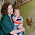## Sunday, January 31, 2016

### 37 49 66 67 121 | Levi's Stadium coordinates, a 'revelation' about the location

Revelation is the 66th Book of the Bible.  This year the 49ers have been in the NFL for 66-years, joining in 1949.  They are hosting Super Bowl 50, a tradition which began at the end of the '66 Season.

Fifty = 6+9+6+20+25 = 66
NFL = 40+6+20 = 66
First Super Bowl at end of '66 season
Revelation is the 66th Book of the Bible
49ers, 66-years old
The Church of Satan was established in SF in '66

Satanic = 19+1+20+1+14+9+3 = 67
Levi's = 12+5+22+9+19 = 67

Anyhow, with all this talk of 'revelation', it has gematria of '121'.  Notice the stadium is on the 121st meridian and the 37th parallel.  These are both interesting numbers, in light of their gematria relationships.

Revelation = 18+5+22+5+12+1+20+9+15+14 = 121
Revelation = 9+5+4+5+3+1+2+9+6+5 = 49/67 (67 with v-exception)

Revelation, is about the end times, and hell coming to earth.

Hell = 8+5+12+12 = 37

Stay tuned.  A lot isn't nice about Levi's Stadium, or the people who run the NFL.

1.2.